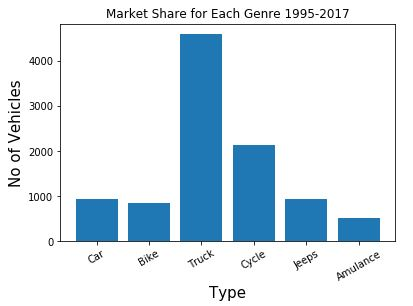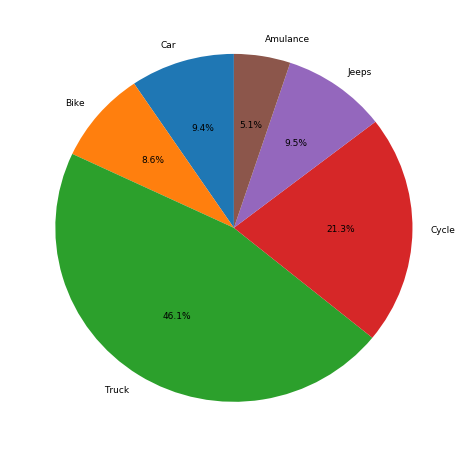# Exploring Categorical Data

Categorical Variable/Data (or Nominal variable):

Such variables take on a fixed and limited number of possible values. For example – grades, gender, blood group type, etc. Also, in the case of categorical variables, the logical order is not the same as categorical data e.g. “one”, “two”, “three”. But the sorting of these variables uses logical order. For example, gender is a categorical variable and has categories – male and female and there is no intrinsic ordering to the categories. A purely categorical variable is one that simply allows you to assign categories, but you cannot clearly order the variables.

Terms related to Variability Metrics :

• Mode : Most frequently occurring value in the given data
Example-

```Data = ["Car", "Bat", "Bat", "Car", "Bat", "Bat", "Bat", "Bike"]
Mode = "Bat"```
• Expected Value : When working in machine learning, categories have to be associated with a numeric value, so as to give understanding to the machine. This gives an average value based on a category’s probability of occurrence i.e. Expected Value.
It is calculated by –

```-> Multiply each outcome by its probability of occurring.
-> Sum these values```

So, it is the sum of values times their probability of occurrence often used to sum up factor variable levels.

•• Bar Charts : Frequency of each category plotted as bars.

 `import` `matplotlib.pyplot as plt ` `import` `numpy as np `

Data –

 `label ``=` `[``'Car'``, ``'Bike'``, ``'Truck'``, ``'Cycle'``, ``'Jeeps'``, ``'Amulance'``] ` `no_vehicle ``=` `[``941``, ``854``, ``4595``, ``2125``, ``942``, ``509``] `

Indexing Data –

 `index ``=` `np.arange(``len``(label)) ` ` `  `print` `(``"Total Labels : "``, ``len``(label)) ` `print` `(``"Indexing : "``, index) `

Output:

```Total Labels :  6
Indexing :  [0 1 2 3 4 5]```

Bar Graph –

 `plt.bar(index, no_vehicle) ` `plt.xlabel(``'Type'``, fontsize ``=` `15``) ` `plt.ylabel(``'No of Vehicles'``, fontsize ``=` `15``) ` `plt.xticks(index, label, fontsize ``=` `10``, rotation ``=` `30``) ` `plt.title(``'Market Share for Each Genre 1995-2017'``) ` ` `  `plt.show() `

Output:• Pie Charts : Frequency of each category plotted as pie or wedges. It is a circular graph, where the arc length of each slice is proportional to the quantity it represents.

 `plt.figure(figsize ``=``(``8``, ``8``)) ` `plt.pie(no_vehicle, labels ``=` `label,  ` `        ``startangle ``=` `90``, autopct ``=``'%.1f %%'``) ` `plt.show() `

Output:My Personal Notes arrow_drop_upIf you like GeeksforGeeks and would like to contribute, you can also write an article using contribute.geeksforgeeks.org or mail your article to contribute@geeksforgeeks.org. See your article appearing on the GeeksforGeeks main page and help other Geeks.

Please Improve this article if you find anything incorrect by clicking on the "Improve Article" button below.

Improved By : nidhi_biet